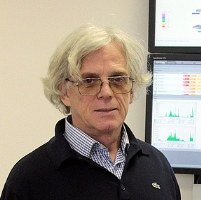Acceptance Rate is a Thermodynamic Function in Local Monte Carlo Algorithms
Speaker
Prof. Lev Shchur
Landau Institute for Theoretical Physics
Abstract

We study properties of Markov chain Monte Carlo simulations of classical spin models with local updates. We derive analytic expressions for the mean value of the acceptance rate of single-spin-flip algorithms for the one-dimensional Ising model. We find that for the Metropolis algorithm the average acceptance rate is a linear function of energy. We further provide numerical results for the energy dependence of the average acceptance rate for the 3- and 4-state Potts model, and the XY model in one and two spatial dimensions. In all cases, the acceptance rate is an almost linear function of the energy in the critical region. The variance of the acceptance rate is studied as a function of the specific heat. While the specific heat develops a singularity in the vicinity of a phase transition, the variance of the acceptance rate stays finite.
Our result for the acceptance rate of the heat-bath algorithm for the one-dimensional Ising model can be viewed as an addition to the Glauber paper on the dynamics of the one-dimensional Ising model. The acceptance rate in the Glauber dynamics is the frequency of the spin flips.